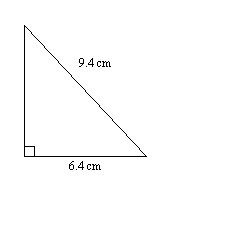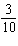Name:    Math 10W Practice Unit 5 Test #4

Multiple Choice
Identify the choice that best completes the statement or answers the question.

1.

A ladder is 5 m long. If it is leaning against a wall, with the bottom of the ladder 1.5 m away from the base of the wall, how high up the wall will it reach?
 a. 2.57 m c. 3.67 m b. 5.22 m d. 4.77 m

2.

The sine ratio relates to which two sides of a right triangle?
 a. The side opposite a given angle and the hypotenuse. b. The side adjacent to a given angle and the hypotenuse. c. The side adjacent to a given angle and the opposite side. d. The side adjacent to a given angle and the vertical side.

3.

What is the sine of 79°?
 a. 0.191 c. 1.016 b. 5.145 d. 0.982

4.

A right triangle has an angle of 8° and the opposite side is 246 cm. What is the length of the hypotenuse?
 a. 248.42 cm c. 1750.38 cm b. 1778.88 cm d. 1767.58 cm

5.

The tangent ratio is ________________ as the angle increases from 0° to 90°.
 a. Mostly increasing, sometimes decreasing c. Always increasing b. Mostly decreasing, sometimes increasing d. Always decreasing

6.

What is the tangent of 40°?
 a. 0.766 c. 0.643 b. 0.839 d. 0.677

7.

A right triangle has an angle of 15°. If the opposite side is 67 cm long, what is the length of the adjacent side?
 a. 250.0 cm c. 253.7 cm b. 258.9 cm d. 254.9 cm

8.

What is sin-1(0.21)?
 a. 12.12° c. 43.32° b. 11.86° d. 77.88°

9.

What is cos-1(0.37)?
 a. 52.92° c. 68.28° b. 21.72° d. 20.30°

10.

The hypotenuse of a right triangle is 20.8 cm and one leg is 4.2 cm long. What is the angle adjacent to the 4.2 cm side?
 a. 11.65° c. 78.35° b. 90.65° d. 11.42°

1.

Solve for the unknown side length.2.

A right triangle has a hypotenuse of 24 m. If one of the angles is 54°, what is the length of the opposite side?

3.

A 8-m long extension cord is needed to power a drill that a worker is using at shoulder level, with the cord fully extended from the plug in the wall. The angle of elevation of the cord is 39.7°. What is the horizontal distance from the plug to where the worker is using the drill?

4.

A mountain is 1300 m tall and its peak is 1774 m up the side of the hill. At what angle does the mountain rise?

5.

In a right triangle, the side adjacent to a given angle isas long as the hypotenuse. What is the measure of the angle?